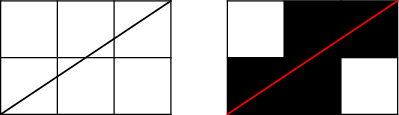시간 제한메모리 제한제출정답맞힌 사람정답 비율
1 초 1024 MB0000.000%

## 문제

Tomas is a computer graphics student. He has a homework which is very easy for him. He has to make a program that draws a line from point (0, 0) to (a, b), where integers a, b (a>0, b> 0) are the input of the program.

He uses the following algorithm. He divides the plane into squares 1x1 – these squares are pixels. When the line from (0, 0) to (a, b) intersects a square in more than one point, the square (pixel) will be black. Otherwise it will be white. Look at the example:Tomas is a computer graphics student. He has a homework which is very easy for him. He has to make a program that draws a line from point (0, 0) to (a, b), where integers a, b (a>0, b> 0) are the input of the program.

He uses the following algorithm. He divides the plane into squares 1x1 – these squares are pixels. When the line from (0, 0) to (a, b) intersects a square in more than one point, the square (pixel) will be black. Otherwise it will be white. Look at the example:

## 입력

The first line of the input file contains an integer T specifying the number of test cases. Each test case is preceded by a blank line.

Each test case looks as follows: The one and only line contains a positive integer N. You can assume that number N has at most 47 divisors.

## 출력

For each test case output one line with one integer – the number of lines that use exactly N black pixels.

• 1 ≤ T ≤ 60

## Easy (1점)

• 1 ≤ N < 10,000

• 1 ≤ N < 1014

## 예제 입력 1

2

2

6


## 예제 출력 1

3
11


## 채점 및 기타 정보

• 예제는 채점하지 않는다.